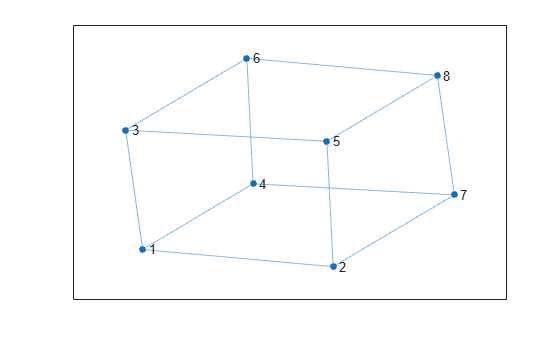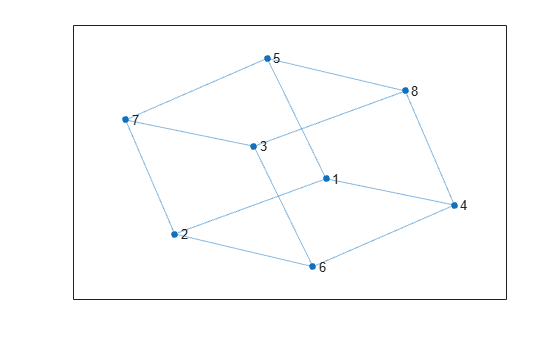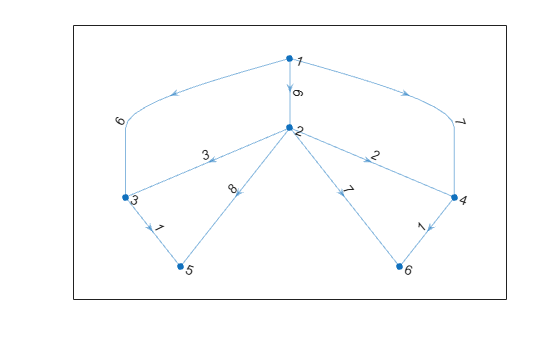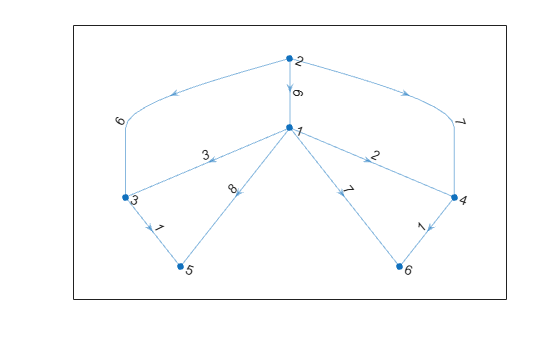# reordernodes

## 语法

``H = reordernodes(G,order)``
``[H,idx] = reordernodes(G,order)``

## 说明

``H = reordernodes(G,order)` 根据 `order` 对图 `G` 中的节点重新排序。此重新排序对应于 `G` 的邻接矩阵的对称置换。`

``[H,idx] = reordernodes(G,order)` 还会返回边索引 `idx` 的置换向量。例如，如果 `G.Edges` 具有变量 `Weight`，则 `H.Edges.Weight == G.Edges.Weight(idx)`。`

## 示例

```s = [1 1 1 2 5 3 6 4 7 8 8 8]; t = [2 3 4 5 3 6 4 7 2 6 7 5]; G = graph(s,t); plot(G)``````order = [7 2 3 4 8 1 5 6]; G = reordernodes(G,order); plot(G)``````s = [1 1 1 2 2 2 2 3 4]; t = [3 4 2 3 4 5 6 5 6]; weights = [6 7 6 3 2 8 7 1 1]; G = digraph(s,t,weights); plot(G,'EdgeLabel',G.Edges.Weight)````[~,order] = sort(outdegree(G),'descend')`
```order = 6×1 2 1 3 4 5 6 ```
```[H,idx] = reordernodes(G,order); plot(H,'EdgeLabel',H.Edges.Weight)````idx` 值描述 `G.Edges` 中行的置换。使用 `Weight` 变量确认此对应关系。

`isequal(H.Edges.Weight, G.Edges.Weight(idx))`
```ans = logical 1 ```

## 输入参数

`order` 可以是以下项之一：

• 节点索引的数值向量，例如 `[1 3 2]`

• 节点名称的字符向量元胞数组或字符串数组，例如 `{'A' 'C' 'B'}``["A" "C" "B"]`

## 输出参数

• `H.Nodes``G.Nodes(order,:)` 相同。

• `H.Edges` 类似于 `G.Edges(idx,:)`，不同的是节点经过重新编号。